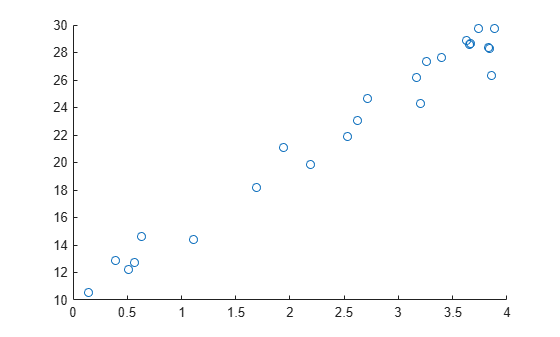# invpred

Inverse prediction

## Syntax

```X0 = invpred(X,Y,Y0) [X0,DXLO,DXUP] = invpred(X,Y,Y0) [X0,DXLO,DXUP] = invpred(X,Y,Y0,name1,val1,name2,val2,...) ```

## Description

`X0 = invpred(X,Y,Y0)` accepts vectors `X` and `Y` of the same length, fits a simple regression, and returns the estimated value `X0` for which the height of the line is equal to `Y0`. The output, `X0`, has the same size as `Y0`, and `Y0` can be an array of any size.

`[X0,DXLO,DXUP] = invpred(X,Y,Y0)` also computes 95% inverse prediction intervals. `DXLO` and `DXUP` define intervals with lower bound `X0–DXLO` and upper bound `X0+DXUP`. Both `DXLO` and `DXUP` have the same size as `Y0`.

The intervals are not simultaneous and are not necessarily finite. Some intervals may extend from a finite value to `-Inf` or `+Inf`, and some may extend over the entire real line.

`[X0,DXLO,DXUP] = invpred(X,Y,Y0,name1,val1,name2,val2,...)` specifies optional argument name/value pairs chosen from the following list. Argument names are case insensitive and partial matches are allowed.

NameValue
`'alpha'`

A value between 0 and 1 specifying a confidence level of `100*(1-alpha)`%. Default is `alpha=0.05` for 95% confidence.

`'predopt'`

Either `'observation'`, the default value to compute the intervals for `X0` at which a new observation could equal `Y0`, or `'curve'` to compute intervals for the `X0` value at which the curve is equal to `Y0`.

## Examples

collapse all

Generate sample data.

```x = 4*rand(25,1); y = 10 + 5*x + randn(size(x));```

Make a scatterplot of the data.

`scatter(x,y)`Predict the x value for a given y value of 20.

`x0 = invpred(x,y,20)`
```x0 = 1.9967 ```